# CAT 2019 Question Paper | Quants Slot 1

###### CAT Previous Year Paper | CAT Quants Questions | Question 29

CAT 2019 did not have a lot of classic mensuration questions that we have seen in CAT previous year papers. However this question was a direct application of the mensuration formulas. Anybody who has done a good CAT Preparation would have been able to tackle this question easily. It deals with the basics of diagnols and sides of quadrilaterals.

Question 29 : The number of solutions of the equation |x|(6x2 + 1) = 5x2 is [TITA]

## Best CAT Coaching in Chennai

#### CAT Coaching in Chennai - CAT 2022Limited Seats Available - Register Now!

|x| (6x2 + 1) = 5x2
We know that |x2| = x2
Let y = |x|
So, y2 = x2
So, y (6y2 + 1) = 5y2
6y2 + 1 = 5y
6y2 -5 + 1 = 0
y =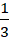or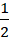Since, y = |x|
y =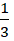or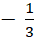,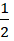or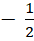Since we have cancelled y in our first step, x = 0 is also a solution
So, Number of possible solutions = 4 + 1 = 5

The question is "The number of solutions of the equation |x|(6x2 + 1) = 5x2 is [TITA] "

##### Hence, the answer is 5

###### CAT Coaching in ChennaiCAT 2023

Classroom Batches Starting Now! @Gopalapuram

###### Best CAT Coaching in Chennai Introductory offer of 5000/-

Attend a Demo Class

##### Where is 2IIM located?

2IIM Online CAT Coaching
A Fermat Education Initiative,
58/16, Indira Gandhi Street,
Kaveri Rangan Nagar, Saligramam, Chennai 600 093

##### How to reach 2IIM?

Mobile: (91) 99626 48484 / 94459 38484
WhatsApp: WhatsApp Now
Email: info@2iim.com